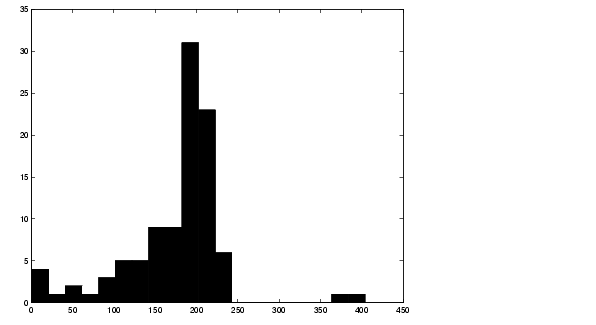Image Processing Toolbox User's Guide11. Compute Statistical Properties of Objects in the Image

Now use MATLAB functions to calculate some statistical properties of the thresholded objects. First use `max` to find the size of the largest grain. (In this example, the largest grain is actually two grains of rice that are touching.)

• ```max([graindata.Area])
```

returns

• ```ans =

404
```

Use the `find` command to return the component label of the grain of rice with this area.

• ```biggrain = find([graindata.Area]==404)
```

returns

• ```biggrain =

59
```

Find the mean of all the rice grain sizes.

• ```mean([graindata.Area])
```

returns

• ```ans =

175.0396
```

Make a histogram containing 20 bins that show the distribution of rice grain sizes. The histogram shows that the most common sizes for rice grains in this image are in the range of 150 to 250 pixels.

• ```hist([graindata.Area],20)```10. Measure Object Properties in the Image Getting Help